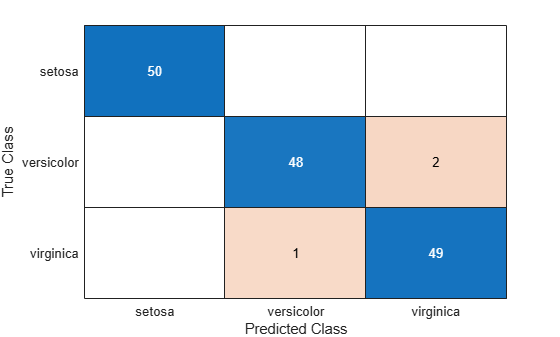# kfoldPredict

Classify observations in cross-validated classification model

## Syntax

``label = kfoldPredict(CVMdl)``
``label = kfoldPredict(CVMdl,'IncludeInteractions',includeInteractions)``
``````[label,Score] = kfoldPredict(___)``````
``````[label,Score,Cost] = kfoldPredict(CVMdl)``````

## Description

example

````label = kfoldPredict(CVMdl)` returns class labels predicted by the cross-validated classifier `CVMdl`. For every fold, `kfoldPredict` predicts class labels for validation-fold observations using a classifier trained on training-fold observations. `CVMdl.X` and `CVMdl.Y` contain both sets of observations.```
````label = kfoldPredict(CVMdl,'IncludeInteractions',includeInteractions)` specifies whether to include interaction terms in computations. This syntax applies only to generalized additive models.```

example

``````[label,Score] = kfoldPredict(___)``` additionally returns the predicted classification scores for validation-fold observations using a classifier trained on training-fold observations, with any of the input argument in the previous syntaxes.```
``````[label,Score,Cost] = kfoldPredict(CVMdl)``` additionally returns the expected misclassification costs for discriminant analysis, k-nearest neighbor, naive Bayes, neural network, and tree classifiers.```

## Examples

collapse all

Create a confusion matrix using the 10-fold cross-validation predictions of a discriminant analysis model.

Load the `fisheriris` data set. `X` contains flower measurements for 150 different flowers, and `y` lists the species, or class, for each flower. Create a variable `order` that specifies the order of the classes.

```load fisheriris X = meas; y = species; order = unique(y)```
```order = 3x1 cell {'setosa' } {'versicolor'} {'virginica' } ```

Create a 10-fold cross-validated discriminant analysis model by using the `fitcdiscr` function. By default, `fitcdiscr` ensures that training and test sets have roughly the same proportions of flower species. Specify the order of the flower classes.

`cvmdl = fitcdiscr(X,y,'KFold',10,'ClassNames',order);`

Predict the species of the test set flowers.

`predictedSpecies = kfoldPredict(cvmdl);`

Create a confusion matrix that compares the true class values to the predicted class values.

`confusionchart(y,predictedSpecies)`Find the cross-validation predictions for a model based on Fisher's iris data.

`load fisheriris`

Train an ensemble of classification trees using AdaBoostM2. Specify tree stumps as the weak learners.

```rng(1); % For reproducibility t = templateTree('MaxNumSplits',1); Mdl = fitcensemble(meas,species,'Method','AdaBoostM2','Learners',t);```

Cross-validate the trained ensemble using 10-fold cross-validation.

`CVMdl = crossval(Mdl);`

Estimate cross-validation predicted labels and scores.

`[elabel,escore] = kfoldPredict(CVMdl);`

Display the maximum and minimum scores of each class.

`max(escore)`
```ans = 1×3 9.3862 8.9871 10.1866 ```
`min(escore)`
```ans = 1×3 0.0018 3.8359 0.9573 ```

## Input Arguments

collapse all

Cross-validated partitioned classifier, specified as a `ClassificationPartitionedModel`, `ClassificationPartitionedEnsemble`, or `ClassificationPartitionedGAM` object. You can create the object in two ways:

• Pass a trained classification model listed in the following table to its `crossval` object function.

• Train a classification model using a function listed in the following table and specify one of the cross-validation name-value arguments for the function.

Flag to include interaction terms of the model, specified as `true` or `false`. This argument is valid only for a generalized additive model (GAM). That is, you can specify this argument only when `CVMdl` is `ClassificationPartitionedGAM`.

The default value is `true` if the models in `CVMdl` (`CVMdl.Trained`) contain interaction terms. The value must be `false` if the models do not contain interaction terms.

Data Types: `logical`

## Output Arguments

collapse all

Predicted class labels, returned as a categorical vector, logical vector, numeric vector, character array, or cell array of character vectors. `label` has the same data type and number of rows as `CVMdl.Y`. Each entry of `label` corresponds to the predicted class label for the corresponding observation in `CVMdl.X`.

If you use a holdout validation technique to create `CVMdl` (that is, if `CVMdl.KFold` is `1`), then ignore the `label` values for training-fold observations. These values match the class with the highest frequency.

Classification scores, returned as an n-by-K matrix, where n is the number of observations (`size(CVMdl.X,1)` when observations are in rows) and K is the number of unique classes (`size(CVMdl.ClassNames,1)`). The classification score `Score(i,j)` represents the confidence that the `i`th observation belongs to class `j`.

If you use a holdout validation technique to create `CVMdl` (that is, if `CVMdl.KFold` is `1`), then `Score` has `NaN` values for training-fold observations.

Expected misclassification costs, returned as an n-by-K matrix, where n is the number of observations (`size(CVMdl.X,1)` when observations are in rows) and K is the number of unique classes (`size(CVMdl.ClassNames,1)`). The value `Cost(i,j)` is the average misclassification cost of predicting that the `i`th observation belongs to class `j`.

Note

If you want to return this output argument, `CVMdl` must be a discriminant analysis, k-nearest neighbor, naive Bayes, neural network, or tree classifier.

If you use a holdout validation technique to create `CVMdl` (that is, if `CVMdl.KFold` is `1`), then `Cost` has `NaN` values for training-fold observations.

## Algorithms

`kfoldPredict` computes predictions as described in the corresponding `predict` object function. For a model-specific description, see the appropriate `predict` function reference page in the following table.

Model Type`predict` Function
Discriminant analysis classifier`predict`
Ensemble classifier`predict`
Generalized additive model classifier`predict`
k-nearest neighbor classifier`predict`
Naive Bayes classifier`predict`
Neural network classifier`predict`
Support vector machine classifier`predict`
Binary decision tree for multiclass classification`predict`

## Version History

Introduced in R2011a

expand all